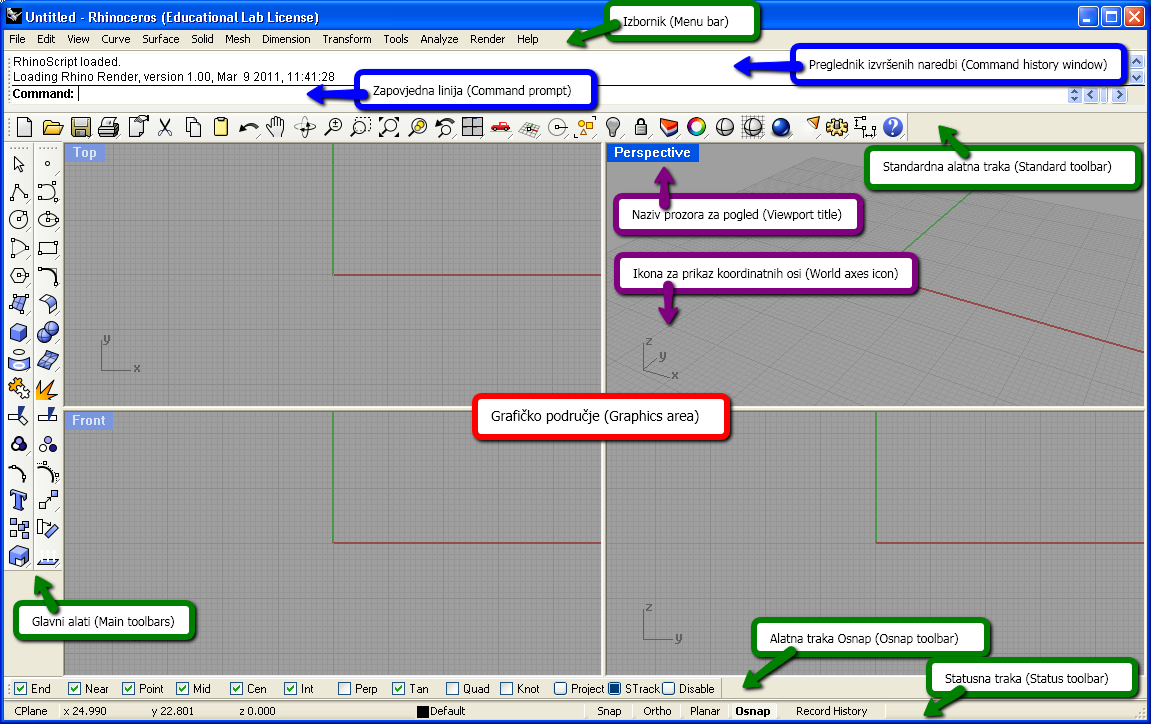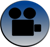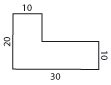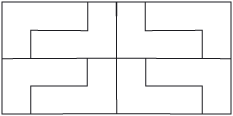# Rhinoceros

 Rhinoceros (Rhino) is a programme for 3D modelling which can be used in various ways and for different purposes. We will use it for modelling plane and spacial objects (solids, surfaces, plane or spacial curves and polygonal lines)as well as for introduction and expansion of the knowledge about their geometrical relations.

## Basics about interface, tabs with view and construction planesFigure 1: Rhino interface with the names pointed out on croatian and english.

 Watch the following two videos in which are explained some of the basic characteristics of the graphic tabs in Rhino (coordinate system, given views in each window, the choice of the method of projection, arrangement of the tabs and the given construction plane), and some basic ways and commands for construction points and line segments. The videos are on croatian language, but the Rhino used has an english interface. If you have already used Rhino, try to solve the exercise 1 by yourself. If this is the first time you are using Rhino, watch the video and try to solve the exercise afterwards. Exercise 1 In each of the three coordinate planes (xy, xz, yz) construct a square with a side length 15 whose edges lie on the positive sides of the coordinate axes.Color these squares in red, blue and green.Construct a cube for which the constructed squares are faces, and translate it for the vector a = -20i-20j-5k.Upper face of the cube color red, front face blue, and right face green. Drawn objects show in the top, front, right view and axonometry.

## Some construction and transformation in the plane

 Exercise 2 Construct a triangle with side 15, 14, and 12 in the xy-plane. Exercise 3 Construct the centroid and orthocenter of a triangle with the side lengths 15, 14 i 12. Exercise 4 Construct the incenter, circumcenter and the Euler line of a triangle with the side lengths 15, 14 i 12.solutionsolutionsolution command line OSNAP used commands: Polyline, Circle tabs LAYERS i PROPERTIES used commands: Polyline, Point, Dimension>Text Block tabs LAYERS i PROPERTIES using commands by typing it into the command line used commands: Polyline, Circle, Point, Dimension>Text Block, Curve>Extend Curve

 Exercise 5 Construct an ellipse in the xy- plane for which the length of the half-axes is 6 and 4, and a center at the origin of the coordinate system. Determine the angle between tangents from the point (8,8) to the ellipse. Construct a tangent to the ellipse at the point of the ellipse for which the coordinates are as follows: x-coordinate (abscissa) equals 4, and y-coordinate (ordinate) is less than 0.solution command line OSNAP (Tan) used commands: Ellipse , Point , Polyline, Dimension>Angle Dimension , Curve>Extend Curve , Curve>Curve From Object>Intersection , Line>Tangent Line From Curve

 Exercise 6 Construct the object given in figure 2 in the xy-plane, and than construct the object in figure 3 using the plane transformations.Figure 2Figure 3solution
used commands: Rectangle , Polyline, Trim , Join , Rotate 2-D , Copy , Move , Mirror

## Some construction and transformation in space

### Surfaces

 Example 1 Construct a part of the plane P(20,15,-10).plohe-ravnina
 Example 2 Surfaces constructed with the command Extrude Curve.plohe-Extrude
window PROPERTIES (Isocurve Density)
used commands: Point, Surface>Plane>3 Points, Surface>Extend Surface, Transform>Scale>Scale 3-D
used commands: Circle, Surface>Extrude Sufrace>Straight (To Point, Along Curve), Analyze>Mass Properties>(Area, Volume), Solid>Cap Planar Holes

### Solids

 Example 3 Modelling of a quadrangle and a sphere.kvadar-kugla-sfera
 Example 4 Modelling of a cone and cylinder.valjak-stožac
used commands:
Solid>Box, Solid>Extrude Planar Curve>Srtaight, Solid>Sphere
used commands:
Solid>Cylinder, Solid>Cone, Extrude Planar Curve>Straight ( Along Curve, To Point)

 Exercise 7 Construct a right circular cone with the base in xy-plane. Center of the base is (15,10,0), radius of the base is 6, and the height of the cone is 17. Construct a right circular cylinder for which the points (15,0,6),(15,20,6) are the center of its bases, and the radius is 4. Using Boolean operations construct the union, difference and intersection of those solids.solution used commands: Solid>Cone, Solid>Cylinder, Copy, Solid>Union, Solid>Intersection, Solid>Difference

 Exercise 8 Construct a cube with the side length 10 and construct the plane where the intersection with the cube is a regular hexagon. Create a solid obtained by the intersection of the cube and the plane. Exercise 9 Construct a regular octahedron with the side length 14.solutionsolution used commands: Solid>Box, Polyline, Surface>Plane>3 Points, Trim, Cap Planar Holes used commands: Polygon, Polyline, Transform>Rotate 3-D, Solid>Pyramid, Transform>Mirror

Created by Sonja Gorjanc and Ema Jurkin- 3DGeomTeh - Developing project of the University of Zagreb
Translated by Helena Halas and Iva Kodrnja